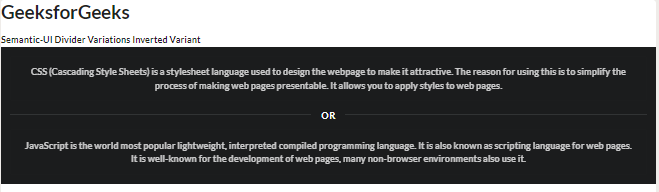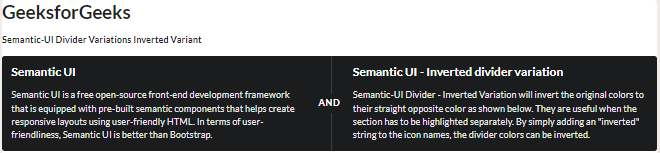# Semantic-UI Divider Variations Inverted Variant

Semantic UI is a free open-source front-end development framework that is equipped with pre-built semantic components that helps create responsive layouts using user-friendly HTML.  It uses predefined CSS and jQuery to incorporate different frameworks.

A Semantic UI divider is generally used to divide the content concisely into different sections. Semantic UI has 3 different types of dividers, each type can have 5 different variations. In this article, let us discuss inverted variations in the divider variations supported in Semantic UI.

Semantic-UI Divider Inverted Variation will invert the original colors to their straight opposite color as shown below. They are useful when the section has to be highlighted separately. By simply adding an “inverted” string to the icon names, the divider colors can be inverted.

Semantic-UI Divider Variations Inverted Variant Class:

• inverted: This class is used to make the divider have its colors inverted.

Syntax:

```<div class ="ui inverted center aligned basic segment">
<div class="ui inverted divider">
....
....
</div>
</div>```

Example 1: The following example demonstrates the inverted variation of the horizontal divider.

## HTML

 ` ` `<``html``> ` ` `  `<``head``> ` `    ``<``link` `href``= ` `"https://cdnjs.cloudflare.com/ajax/libs/semantic-ui/2.4.1/semantic.min.css"`  `          ``rel``=``"stylesheet"` `/>  ` `    `` ` ` `  `<``body``> ` `    ``<``h1``>GeeksforGeeks  ` `    ``<``strong``>Semantic-UI Divider Variations Inverted Variant ` `    ``<``div` `class``=``"ui inverted center aligned basic segment"``> ` `        ``<``div` `class``=``"ui inverted message"``> ` `            ``<``p``> ` `                ``CSS (Cascading Style Sheets) is a stylesheet ` `                ``language used to design the webpage to make ` `                ``it attractive. The reason for using this is  ` `                ``to simplify the process of making web pages ` `                ``presentable. It allows you to apply styles ` `                ``to web pages.  ` `            `` ` ` `  `        `` ` `        ``<``div` `class``=``"ui inverted horizontal divider"``> Or ` `        ``<``div` `class``=``"ui inverted message"``> ` `            ``<``p``> ` `                ``JavaScript is the world most popular lightweight, ` `                ``interpreted compiled programming language. It is  ` `                ``also known as scripting language for web pages.  ` `                ``It is well-known for the development of web pages, ` `                ``many non-browser environments also use it.  ` `            `` ` ` `  `        `` ` `    `` ` ` ` ` `  ``

Output:Semantic-UI Divider Variations Inverted Variant

Example 2: In the below example, we can see an inverted divider variation using a vertical divider, which divides two sections into inverted vertical sections.

## HTML

 ` ` `<``html``> ` ` `  `<``head``> ` `    ``<``link` `href``= ` `"https://cdnjs.cloudflare.com/ajax/libs/semantic-ui/2.4.1/semantic.min.css"`  `          ``rel``=``"stylesheet"` `/> ` ` ` ` `  `<``body``> ` `    ``<``h1``>GeeksforGeeks ` `    ``<``strong``>Semantic-UI Divider Variations Inverted Variant ` `    ``<``div` `class``=``"ui inverted segment"``> ` `        ``<``div` `class``=``"ui inverted two column very relaxed grid"``> ` `            ``<``div` `class``=``"column"``> ` `                ``<``h3` `class``=``"ui inverted header"``>  ` `                    ``Semantic UI  ` `                `` ` `                ``<``p``> ` `                    ``Semantic UI is a free open-source front-end ` `                    ``development framework that is equipped with ` `                    ``pre-built semantic components that helps create ` `                    ``responsive layouts using user-friendly HTML. In  ` `                    ``terms of user-friendliness, Semantic UI is better ` `                    ``than Bootstrap. ` `                `` ` ` `  `            `` ` `            ``<``div` `class``=``"column"``> ` `                ``<``h3` `class``=``"ui inverted header"``>  ` `                    ``Semantic UI - Inverted divider variation ` `                `` ` `                ``<``p``> ` `                    ``Semantic-UI Divider - Inverted Variation will  ` `                    ``invert the original colors to their straight  ` `                    ``opposite color as shown below. They are useful ` `                    ``when the section has to be highlighted separately. ` `                    ``By simply adding an "inverted" string to the icon ` `                    ``names, the divider colors can be inverted. ` `                `` ` ` `  `            `` ` `        `` ` `        ``<``div` `class``=``"ui inverted vertical divider"``>AND ` `    `` ` ` ` ` `  ``

Output:Semantic-UI Divider Variations Inverted Variant

Whether you're preparing for your first job interview or aiming to upskill in this ever-evolving tech landscape, GeeksforGeeks Courses are your key to success. We provide top-quality content at affordable prices, all geared towards accelerating your growth in a time-bound manner. Join the millions we've already empowered, and we're here to do the same for you. Don't miss out - check it out now!

Previous
Next